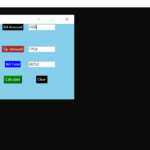## Tip Calculator In Python with Source Code

The Tip Calculator In Python is a simple project developed using Python. The project is for the user convenient, for calculating the tip. This project is an interesting project. The user can calculate the tip amount that they can get from a single bill pa...

Type : Project

File Size : 911 bytes

Please click the button below to download the ZIP File.# Similar Projects and Reports

#### Tip Calculator In Python with Source Code

The Tip Calculator In Python is a simple project developed using Python. The project is for the user convenient, for calculating the tip. This project is an interesting project. The user can calculate the tip amount that they can get from a single bill pa...

#### CALCULATOR IN PYTHON WITH SOURCE CODE

This Calculator project In Python is a simple project developed using Python. The project contains the numbers, operators, and sign like in normal calculator. So, the user can enter any number they want in the calculations. Hence, the user can use a simpl...

#### GUI CALCULATOR IN PYTHON WITH SOURCE CODE

The GUI Calculator In Python is a simple project developed using Python. The project contains the numbers, operators, and sign like in normal calculator. So, the user cannot enter the number themselves, they can just click on the numbers they want in the ...

#### BMI Calculator In Python With Source Code

The BMI Calculator In Python is a simple project developed using Python. The project is for the user convenient, for checking your BMI. With the BMI value, you can check whether you have a healthy weight or not. The project file contains a python script ...

#### Age Calculator In Python With Source Code

The Age Calculator In Python is a simple project developed using Python. The project is for the user convenient, to help them find out about their exact age in year, month and days. The project file contains a python script ( Age_Calculator_GUI.py). This ...

#### Calculator With GUI In Python With Source Code

The Calculator With GUI In Python is a simple project developed using Python. The project contains the numbers, operators, and signs like in a normal calculator. So, the user cannot enter the number themselves, they can just click on the numbers they want...

Visit code-projects.org to download thousands of other free projects